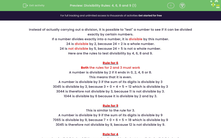# Know the Rules for Dividing 4, 6, 8 and 9

In this worksheet, students will use the rules for dividing by 4, 6, 8 and 9.Key stage:  KS 3

Curriculum topic:   Number

Curriculum subtopic:   Use Concepts and Vocabulary for All Numbers

Difficulty level:#### Worksheet Overview

Instead of actually carrying out a division, it is possible to test a number to see if it can be divided exactly by certain numbers.

If a number divides exactly into a number, it is divisible by this number.

24 is divisible by 2 because 24 ÷ 2 is a whole number.

24 is not divisible by 5 because 24 ÷ 5 is not a whole number.

Here are the rules to test divisibility by 4, 6, 8 and 9.

Rule for 6

Both the rules for 2 and 3 must work

A number is divisible by 2 if it ends in 0, 2, 4, 6 or 8.

This means that it must be even.

A number is divisible by 3 if the sum of its digits is divisible by 3.

3045 is divisible by 3 because 3 + 0 + 4 + 5 = 12 which is divisible by 3.

3044 is therefore not divisible by 3 because 11 is not divisible by 3.

1044 is divisible by 6 because it is divisible by 2 and by 3.

Rule for 9

This is similar to the rule for 3.

A number is divisible by 9 if the sum of its digits is divisible by 9.

7065 is divisible by 9 because 7 + 0 + 6 + 5 = 18 which is divisible by 9.

3045 is therefore not divisible by 9 because 12 is not divisible by 9.

Rule for 4

A number is divisible by 4 if the number formed by its last two digits is divisible by 4.

3140 is divisible by 4 because 40 is divisible by 4.

3145 is not divisible by 4 because 45 is not divisible by 4.

Rule for 8

A number is divisible by 8 if the number formed by its last three digits is divisible by 8.

3240 is divisible by 8 because 240 is divisible by 8.

3145 is not divisible by 8 because 145 is not divisible by 8.

Example

Is 3813 divisible by 6?

No, because although 3 + 8 + 1 + 3 = 15 is divisible by 3, it is not divisible by 2 as it ends in a 3, which is an odd number.

Example

Is 2,153,864 divisible by 4?

Yes because 64 is divisible by 4.

Have you got the hang of this?Let's have a go at some questions. If you get stuck, you can look back to this page by clicking on the pink button at the side of the screen.

### What is EdPlace?

We're your National Curriculum aligned online education content provider helping each child succeed in English, maths and science from year 1 to GCSE. With an EdPlace account you’ll be able to track and measure progress, helping each child achieve their best. We build confidence and attainment by personalising each child’s learning at a level that suits them.

Get started